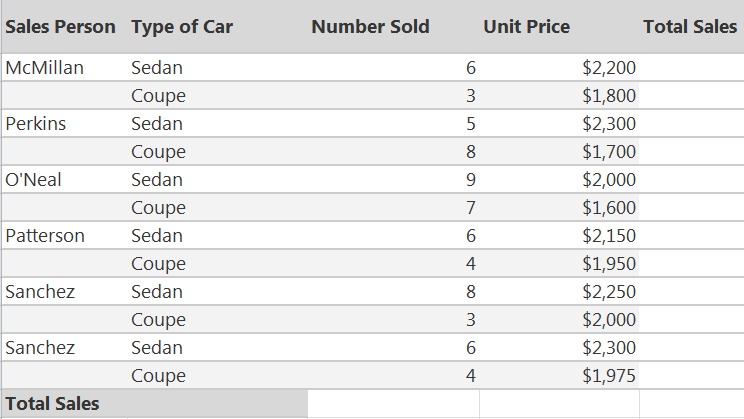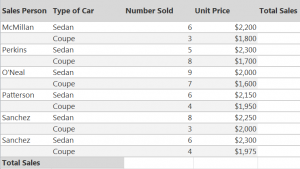# How to Create Arrays in Excel6
JanThe array formulas for Excel work to perform more complicated calculations than basic formulas. The primary purpose of array formulas is to calculate numbers that meet a specific set of conditions and work with a wide range of cells.

In the example, you will use array formulas with a car sales report spreadsheet.

## The Multi-Cell Array Formula for Excel

To begin, multiply the values of the array in the cell ranges of C2 through D13. This is the number sold multiplied by the unit price for each individual row. When using multi-cell arrays, you are able to write the formula in one place and have it populate other cells that you choose.

For this formula, select cells E2 through E13 then type this in the formula bar:

=C2:C13*D2:D13

Press CTRL + SHIFT + ENTER and with this combination of keys, you let Excel know that you are entering an array formula. Your formula then be surrounded with curly braces in the formula bar as a response.

If  you have entered the array formula correctly, all cells will fill with the correct answer.

## The Single Cell Array Formula for Excel

Now you are able to calculate the total number of sales. Select cell B14 and enter the following formula to add together the products of “number sold” and “unit price” columns.

=SUM(C2:C13*D2:D13)

Press CTRL + SHIFT + ENTER to enter the formula as an array. The answer will appear, and you are finished with the tutorial.